List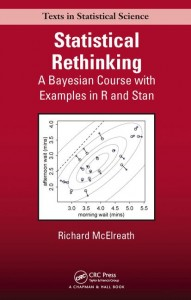Here I work through the practice questions in Chapter 5, “Multivariate Linear Models,” of Statistical Rethinking (McElreath, 2016). I do my best to use only approaches and functions discussed so far in the book, as well as to name objects consistently with how the book does. If you find any typos or mistakes in my answers, or if you have any relevant questions, please feel free to add a comment below.

Here is the chapter summary from page 162:

This chapter introduced multiple regression, a way of constructing descriptive models for how the mean of a measurement is associated with more than one predictor variable. The defining question of multiple regression is: What is the value of knowing each predictor, once we already know the other predictors? Implicit in this question are: (1) a focus on the value of the predictors for description of the sample, instead of forecasting a future sample; and (2) the assumption that the value of each predictor does not depend upon the values of the other predictors. In the next two chapters, we confront these two issues.

# Setup
library(rethinking)
data("WaffleDivorce")
data("foxes")
set.seed(5)


## Easy Difficulty

### Practice Question 5E1

Which of the linear models below are multiple regressions?

(1) $$\mu_i = \alpha + \beta x_i$$

(2) $$\mu_i = \beta_x x_i + \beta_z z_i$$

(3) $$\mu_i = \alpha + \beta(x_i – z_i)$$

(4) $$\mu_i = \alpha + \beta_x x_i + \beta_z z_i$$

The first model has only one predictor, $$x_i$$, so is not a multiple regression. The second model has two predictors, $$x_i$$ and $$z_i$$, so is a multiple regression (despite having no intercept). The third model is tricky because it includes two predictor variables but only one slope term; however, algebra would apply the multiplication of $$\beta$$ to both $$x_i$$ and $$z_i$$, so we can consider this to be a strangely formatted multiple regression in which the slope for $$z_i$$ is constrained to be equal to $$-1$$ times the slope for $$x_i$$. The fourth model has two predictors, $$x_i$$ and $$z_i$$, so is a multiple regression.

Thus, models 2, 3, and 4 are multiple regressions.

### Practice Question 5E2

Write down a multiple regression to evaluate the claim: Animal diversity is linearly related to latitude, but only after controlling for plant diversity. You just need to write down the model definition.

The wording of the question makes it unclear which of the first two variables is the outcome and which is the predictor, but I will assume that latitude is the outcome (so as to keep the two diversity variables together).
$\mu_i = \alpha + \beta_A A_i + \beta_P P_i$
where $$A$$ is animal diversity and $$P$$ is plant diversity.

### Practice Question 5E3

Write down a multiple regression to evaluate the claim: Neither the amount of funding nor size of laboratory is by itself a good predictor of time to PhD degree; but together these variables are both positively associated with time to degree. Write down the model definition and indicate which side of zero each slope parameter should be on.

I will provide the linear model in math form (page 139):
$\mu_i = \alpha + \beta_F F_i + \beta_S S_i$
where $$F$$ is amount of funding and $$S$$ is size of laboratory.

Given the question text, both slope parameters, $$\beta_f$$ and $$\beta_s$$, should be positive.

### Practice Question 5E4

Suppose you have a single categorical predictor with 4 levels (unique values), labeled A, B, C, and D. Let $$A_i$$ be an indicator variable that is 1 where case $$i$$ is in category A. Also suppose $$B_i$$, $$C_i$$, and $$D_i$$ for the other categories. Now which of the following linear models are inferentially equivalent ways to include the categorical variable in a regression? Models are inferentially equivalent when it’s possible to compute one posterior distribution from the posterior distribution of another model.

(1) $$\mu_i = \alpha + \beta_A A_i + \beta_B B_i + \beta_D D_i$$

(2) $$\mu_i = \alpha + \beta_A A_i + \beta_B B_i + \beta_C C_i + \beta_D D_i$$

(3) $$\mu_i = \alpha + \beta_B B_i + \beta_C C_i + \beta_D D_i$$

(4) $$\mu_i = \alpha_A A_i + \alpha_B B_i + \alpha_C C_i + \alpha_D D_i$$

(5) $$\mu_i = \alpha_A (1 – B_i – C_i – D_i) + \alpha_B B_i + \alpha_C C_i + \alpha_D D_i$$

The first model includes a single intercept (for category C) and slopes for A, B, and D.

The second model is non-identifiable because it includes a slope for all possible categories (page 156).

The third model includes a single intercept (for category A) and slopes for B, C, and D.

The fourth model uses the unique index approach to provide a separate intercept for each category (and no slopes).

The fifth model uses the reparameterized approach on pages 154 and 155 to multiply the intercept for category A times 1 when in category A and times 0 otherwise.

Models 1, 3, 4, and 5 are inferentially equivalent because they each allow the computation of each other’s posterior distribution (e.g., each category’s intercept and difference from each other category).

## Medium Difficulty

### Practice Question 5M1

Invent your own example of a spurious correlation. An outcome variable should be correlated with both predictor variables. But when both predictors are entered in the same model, the correlation between the outcome and one of the predictors should mostly vanish (or at least be greatly reduced).

I will simulate data using the approach from page 135 to invent an example of a spurious correlation between the number of operas seen and score on a standardized achievement test that vanishes when family income is included.

N <- 100
income <- rnorm(n = 100, mean = 0, sd = 1)
operas <- rnorm(n = N, mean = income, sd = 2)
scores <- rnorm(n = N, mean = income, sd = 1)
d <- data.frame(scores, operas, income)
pairs(d)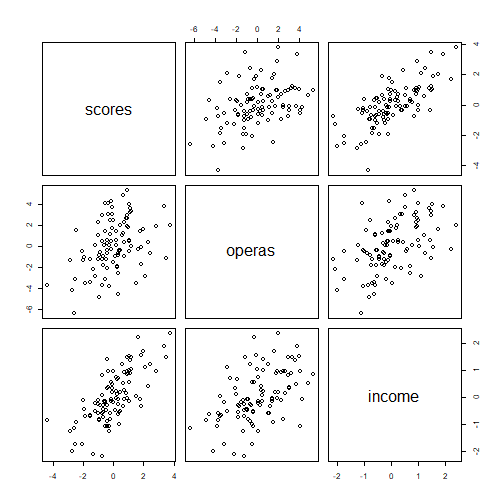Let’s first show that there is a (spurious) association between number of operas seen and score.

m <- map(
alist(
scores ~ dnorm(mu, sigma),
mu <- a + bo * operas,
a ~ dnorm(0, 5),
bo ~ dnorm(0, 5),
sigma ~ dunif(0, 5)
),
data = d
)
precis(m)

##       Mean StdDev  5.5% 94.5%
## a     0.02   0.13 -0.19  0.22
## bo    0.23   0.05  0.14  0.31
## sigma 1.27   0.09  1.12  1.41


Now let’s show that this association vanishes when family income is added to the model.

m <- map(
alist(
scores ~ dnorm(mu, sigma),
mu <- a + bo * operas + bi * income,
a ~ dnorm(0, 5),
bo ~ dnorm(0, 5),
bi ~ dnorm(0, 5),
sigma ~ dunif(0, 5)
),
data = d
)
precis(m)

##       Mean StdDev  5.5% 94.5%
## a     0.00   0.10 -0.16  0.15
## bo    0.03   0.05 -0.05  0.10
## bi    1.03   0.12  0.84  1.21
## sigma 0.95   0.07  0.84  1.06


So, in this model, family income predicts scores on standardized achievement tests when the number of operas seen is already known, but the number of operas seen does not predict scores on standardized achievement tests when family income is already known. Thus, the bivariate association between test scores and exposure to opera is spurious.

### Practice Question 5M2

Invent your own example of a masked relationship. An outcome variable should be correlated with both predictor variables, but in opposite directions. And the two predictor variables should be correlated with one another.

I will use the approach from page 141 to invent an example of a masked relationship involving the prediction of happiness ratings from the amount of alcohol one drinks and the amount of time one spends feeling ill.

N <- 100
rho <- 0.6
alcohol <- rnorm(n = N, mean = 0, sd = 1)
illness <- rnorm(n = N, mean = rho * alcohol, sd = sqrt(1 - rho^2))
happiness <- rnorm(n = N, mean = alcohol - illness, sd = 1)
d <- data.frame(happiness, alcohol, illness)
pairs(d)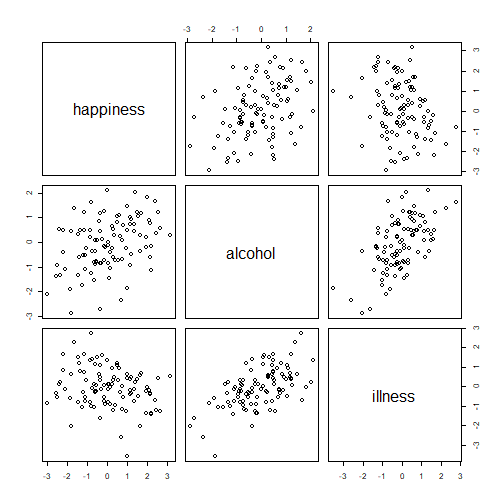Due to the masking relationship, the bivariate associations should be weak/widely variable.

m <- map(
alist(
happiness ~ dnorm(mu, sigma),
mu <- a + ba * alcohol,
a ~ dnorm(0, 5),
ba ~ dnorm(0, 5),
sigma ~ dunif(0, 5)
),
data = d
)
precis(m)

##       Mean StdDev  5.5% 94.5%
## a     0.12   0.13 -0.08  0.32
## ba    0.50   0.12  0.31  0.70
## sigma 1.26   0.09  1.12  1.41

m <- map(
alist(
happiness ~ dnorm(mu, sigma),
mu <- a + bi * illness,
a ~ dnorm(0, 5),
bi ~ dnorm(0, 5),
sigma ~ dunif(0, 5)
),
data = d
)
precis(m)

##        Mean StdDev  5.5% 94.5%
## a      0.06   0.13 -0.15  0.28
## bi    -0.32   0.13 -0.53 -0.11
## sigma  1.33   0.09  1.18  1.48


But in the multivariate regression, the masking relationship should be resolved.

m <- map(
alist(
happiness ~ dnorm(mu, sigma),
mu <- a + ba * alcohol + bi * illness,
a ~ dnorm(0, 5),
ba ~ dnorm(0, 5),
bi ~ dnorm(0, 5),
sigma ~ dunif(0, 5)
),
data = d
)
precis(m)

##        Mean StdDev  5.5% 94.5%
## a      0.11   0.10 -0.05  0.27
## ba     1.04   0.12  0.85  1.23
## bi    -0.95   0.12 -1.14 -0.75
## sigma  1.00   0.07  0.89  1.11


Indeed, the slopes for alcohol and illness became much larger in magnitude in the multivariate model. Thus, in this example (and maybe not in real life), because alcohol increases happiness and feelings of illness, and feelings of illness decrease happiness, the bivariate relationships of alcohol and feelings of illness to happiness are masked.

### Practice Question 5M3

It is sometimes observed that the best predictor of fire risk is the presence of firefighters–States and localities with many firefighters also have more fires. Presumably firefighters do not cause fires. Nevertheless, this is not a spurious correlation. Instead fires cause firefighters. Consider the same reversal of causal inference in the context of the divorce and marriage data. How might a high divorce rate cause a higher marriage rate? Can you think of a way to evaluate this relationship, using multiple regression?

We might hypothesize that a high divorce rate causes a higher marriage rate by introducing more unmarried individuals (who have a demonstrated willingness to marry) into the dating pool. This possibility could be evaluated using multiple regression by regressing marriage rate on both divorce rate and re-marriage rate (i.e., the rate of non-first marriages or marriages following divorces). If divorce rate no longer predicts marriage rate even when the re-marriage rate is known, this would support our hypothesis.

### Practice Question 5M4

In the divorce data, States with high numbers of Mormons (members of The Church of Jesus Christ of Latter-day Saints, LDS) have much lower divorce rates than the regression model expected. Find a list of LDS population by State and use those numbers as a predictor variable, predicting divorce rate using marriage rate, median age at marriage, and percent LDS population (possibly standardized). You may want to consider transformations of the raw percent LDS variable.

I will pull up the percent LDS population by State from Wikipedia and add it as a new column in the WaffleDivorce data frame (minus Nevada as it is apparently missing from WaffleDivorce). Given the positive skew of these percentages, I will log-transform them prior to standardization.

d <- WaffleDivorce
d$LDS <- c(0.0077, 0.0453, 0.0610, 0.0104, 0.0194, 0.0270, 0.0044, 0.0057, 0.0041, 0.0075, 0.0082, 0.0520, 0.2623, 0.0045, 0.0067, 0.0090, 0.0130, 0.0079, 0.0064, 0.0082, 0.0072, 0.0040, 0.0045, 0.0059, 0.0073, 0.0116, 0.0480, 0.0130, 0.0065, 0.0037, 0.0333, 0.0041, 0.0084, 0.0149, 0.0053, 0.0122, 0.0372, 0.0040, 0.0039, 0.0081, 0.0122, 0.0076, 0.0125, 0.6739, 0.0074, 0.0113, 0.0390, 0.0093, 0.0046, 0.1161) d$logLDS <- log(d$LDS) d$logLDS.s <- (d$logLDS - mean(d$logLDS)) / sd(d$logLDS) simplehist(d$LDS)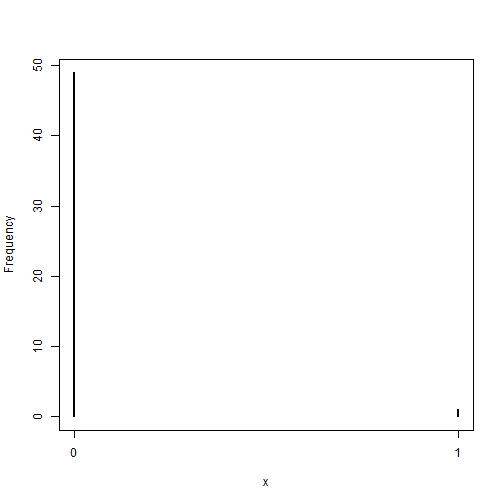simplehist(d$logLDS)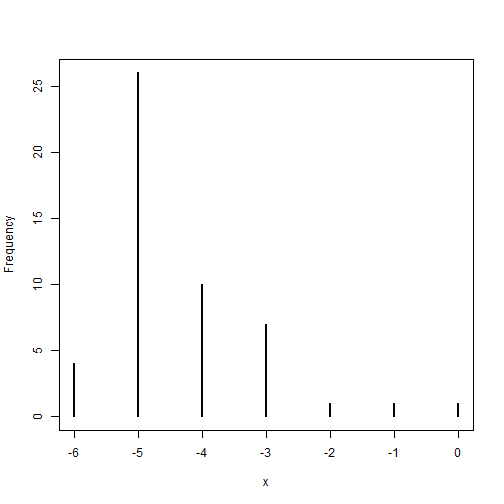simplehist(d$logLDS.s)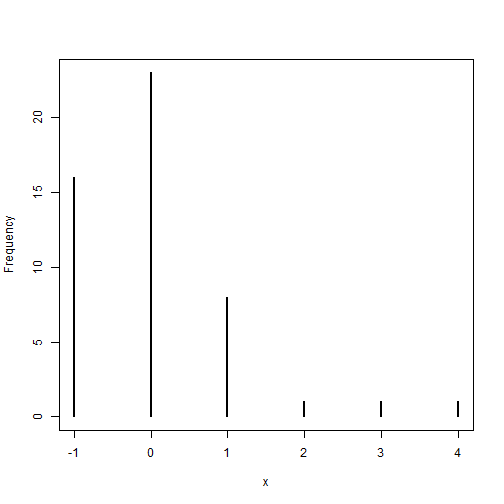The transformed and standardized variables look much better in terms of distribution. Now we can build the requested multiple regression model.

m <- map(
alist(
Divorce ~ dnorm(mu, sigma),
mu <- a + bm * Marriage + ba * MedianAgeMarriage + bl * logLDS.s,
a ~ dnorm(10, 20),
bm ~ dnorm(0, 10),
ba ~ dnorm(0, 10),
bl ~ dnorm(0, 10),
sigma ~ dunif(0, 5)
),
data = d
)
precis(m)

##        Mean StdDev  5.5% 94.5%
## a     35.43   6.78 24.61 46.26
## bm     0.05   0.08 -0.08  0.19
## ba    -1.03   0.22 -1.39 -0.67
## bl    -0.61   0.29 -1.07 -0.14
## sigma  1.38   0.14  1.16  1.60


In this model, the slope of marriage was widely variable and its interval includes zero. However, the slopes of both median age at marriage and percentage of LDS population were negative and their intervals did not include zero. Thus, states with older median age at marriage or higher percentages of Mormons had lower divorce rates.

### Practice Question 5M5

One way to reason through multiple causation hypotheses is to imagine detailed mechanisms through which predictor variables may influence outcomes. For example, it is sometimes argued that the price of gasoline (predictor variable) is positively associated with lower obesity rates (outcome variable). However, there are at least two important mechanisms by which the price of gas could reduce obesity. First, it could lead to less driving and therefore more exercise. Second, it could lead to less driving, which leads to less eating out, which leads to less consumption of huge restaurant meals. Can you outline one or more multiple regressions that address these two mechanisms? Assume you can have any predictor data you need.

To address these two mechanisms, we need variables that would capture them. For the first mechanism, a variable corresponding to time spent exercising would be a reasonable start. For the second mechanism, a variable corresponding to frequency of eating out at restaurant would be a reasonable start. We could get even fancier and try to measure things like calories burned during exercise and calories ingested at restaurants. However, we would want to be careful not to introduce multicollinearity by adding highly correlated variables. So, I would propose the following multiple regression model:
$\mu_i = \alpha + \beta_G G_i + \beta_E E_i + \beta_R R_i$
where $$G$$ represents the price of gasoline, $$E$$ represents one exercise-related variable, and $$R$$ represents one restaurant-related variable. One version of this model might use self-reported frequencies of exercise and eating out, and another version might use more rigorously measured calories burned through exercise and calories ingested from restaurants.

## Hard Difficulty

All three exercises below use the same data, data(foxes) (part of rethinking). The urban fox (Vulpes vulpes) is a successful exploiter of human habitat. Since urban foxes move in packs and defend territories, data on habitat quality and population density is also included. The data frame has five columns:

(1) group: Number of the social group the individual fox belongs to

(2) avgfood: The average amount of food available in the territory

(3) groupsize: The number of foxes in the social group

(4) area: Size of the territory

(5) weight: Body weight of the individual fox

### Practice Question 5H1

Fit two bivariate Gaussian regressions, using map(): (1) body weight as a linear function of territory size, and (2) body weight as a linear function of group size. Plot the results of these regressions, displaying the MAP regression line and the 95% interval of the mean. Is either variable important for predicting fox body weight?

d <- foxes
ma <- map(
alist(
weight ~ dnorm(mu, sigma),
mu <- a + ba * area,
a ~ dnorm(5, 5),
ba ~ dnorm(0, 5),
sigma ~ dunif(0, 5)
),
data = d
)
precis(ma)

##       Mean StdDev  5.5% 94.5%
## a     4.45   0.39  3.83  5.08
## ba    0.02   0.12 -0.16  0.21
## sigma 1.18   0.08  1.06  1.30

area.seq <- seq(from = min(d$area), to = max(d$area), length.out = 30)
mu <- link(ma, data = data.frame(area = area.seq))

## [ 100 / 1000 ]
[ 200 / 1000 ]
[ 300 / 1000 ]
[ 400 / 1000 ]
[ 500 / 1000 ]
[ 600 / 1000 ]
[ 700 / 1000 ]
[ 800 / 1000 ]
[ 900 / 1000 ]
[ 1000 / 1000 ]

mu.PI <- apply(mu, 2, PI, prob = 0.95)
plot(weight ~ area, data = d, col = rangi2)
abline(ma)

## Warning in abline(ma): only using the first two of 3 regression
## coefficients

shade(mu.PI, area.seq)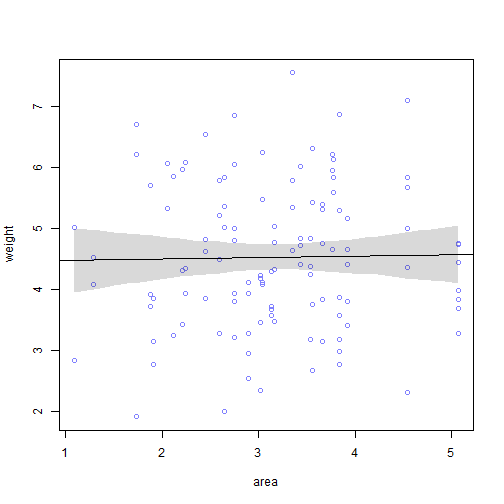Now we can do the same for group size:

mg <- map(
alist(
weight ~ dnorm(mu, sigma),
mu <- a + bg * groupsize,
a ~ dnorm(5, 5),
bg ~ dnorm(0, 5),
sigma ~ dunif(0, 5)
),
data = d
)
precis(mg)

##        Mean StdDev  5.5% 94.5%
## a      5.07   0.32  4.55  5.59
## bg    -0.12   0.07 -0.24 -0.01
## sigma  1.16   0.08  1.04  1.29

groupsize.seq <- seq(from = min(d$groupsize), to = max(d$groupsize), length.out = 30)
mu <- link(mg, data = data.frame(groupsize = groupsize.seq))

## [ 100 / 1000 ]
[ 200 / 1000 ]
[ 300 / 1000 ]
[ 400 / 1000 ]
[ 500 / 1000 ]
[ 600 / 1000 ]
[ 700 / 1000 ]
[ 800 / 1000 ]
[ 900 / 1000 ]
[ 1000 / 1000 ]

mu.PI <- apply(mu, 2, PI, prob = 0.95)
plot(weight ~ groupsize, data = d, col = rangi2)
abline(mg)

## Warning in abline(mg): only using the first two of 3 regression
## coefficients

shade(mu.PI, groupsize.seq)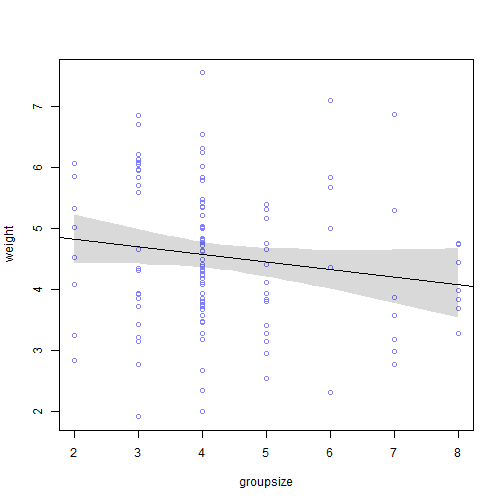Based on only the bivariate regressions, it appears that neither territory area nor group size are very important for the prediction of body weight. Group size seemed like it may have a slightly negative relationship with body weight, but nothing too precise.

### Practice Question 5H2

Now fit a multiple linear regression with weight as the outcome and both area and groupsize as predictor variables. Plot the predictions of the model for each predictor, holding the other predictor constant at its mean. What does this model say about the importance of each variable? Why do you get different results than you got in the exercise just above?

Let’s begin with the regression model before moving on to the counterfactual plots:

mag <- map(
alist(
weight ~ dnorm(mu, sigma),
mu <- a + ba * area + bg * groupsize,
a ~ dnorm(5, 5),
ba ~ dnorm(0, 5),
bg ~ dnorm(0, 5),
sigma ~ dunif(0, 5)
),
data = d
)
precis(mag)

##        Mean StdDev  5.5% 94.5%
## a      4.45   0.37  3.86  5.04
## ba     0.62   0.20  0.30  0.94
## bg    -0.43   0.12 -0.62 -0.24
## sigma  1.12   0.07  1.00  1.24


Now let’s create a counterfactual plot for territory area (page 131)

G.avg <- mean(d$groupsize) A.seq <- seq(from = 0, to = 6, length.out = 30) pred.data <- data.frame( groupsize = G.avg, area = A.seq ) mu <- link(mag, data = pred.data)  ## [ 100 / 1000 ] [ 200 / 1000 ] [ 300 / 1000 ] [ 400 / 1000 ] [ 500 / 1000 ] [ 600 / 1000 ] [ 700 / 1000 ] [ 800 / 1000 ] [ 900 / 1000 ] [ 1000 / 1000 ]  mu.mean <- apply(mu, 2, mean) mu.PI <- apply(mu, 2, PI, prob = 0.95) A.sim <- sim(mag, data = pred.data, n = 1e4)  ## [ 1000 / 10000 ] [ 2000 / 10000 ] [ 3000 / 10000 ] [ 4000 / 10000 ] [ 5000 / 10000 ] [ 6000 / 10000 ] [ 7000 / 10000 ] [ 8000 / 10000 ] [ 9000 / 10000 ] [ 10000 / 10000 ]  A.PI <- apply(A.sim, 2, PI) plot(weight ~ area, data = d, type = "n") mtext("groupsize = 4.345") lines(A.seq, mu.mean) shade(mu.PI, A.seq) shade(A.PI, A.seq)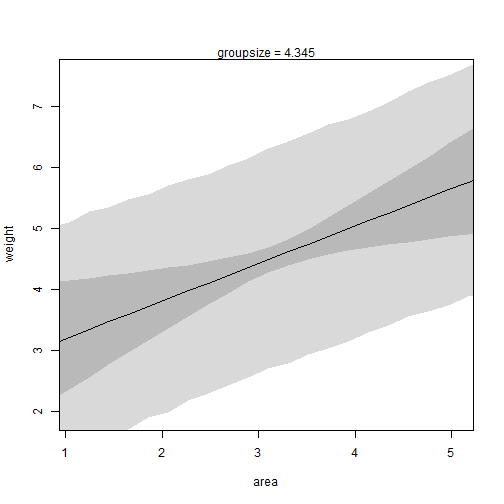We can use the same approach to create a counterfactual plot for group size: A.avg <- mean(d$area)
G.seq <- seq(from = 1, to = 10, length.out = 30)
pred.data <- data.frame(
groupsize = G.seq,
area = A.avg
)
mu <- link(mag, data = pred.data)

## [ 100 / 1000 ]
[ 200 / 1000 ]
[ 300 / 1000 ]
[ 400 / 1000 ]
[ 500 / 1000 ]
[ 600 / 1000 ]
[ 700 / 1000 ]
[ 800 / 1000 ]
[ 900 / 1000 ]
[ 1000 / 1000 ]

mu.mean <- apply(mu, 2, mean)
mu.PI <- apply(mu, 2, PI, prob = 0.95)
G.sim <- sim(mag, data = pred.data, n = 1e4)

## [ 1000 / 10000 ]
[ 2000 / 10000 ]
[ 3000 / 10000 ]
[ 4000 / 10000 ]
[ 5000 / 10000 ]
[ 6000 / 10000 ]
[ 7000 / 10000 ]
[ 8000 / 10000 ]
[ 9000 / 10000 ]
[ 10000 / 10000 ]

G.PI <- apply(G.sim, 2, PI)
plot(weight ~ groupsize, data = d, type = "n")
mtext("area = 3.169")
lines(G.seq, mu.mean)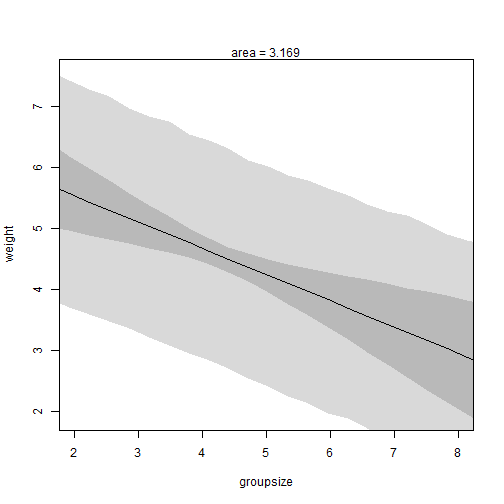The multiple regression model tells a different story than the bivariate models. It says that territory area is positively related to body weight and that group size is negatively related to body weight. The results differ because this is an example of a masking relationship. Territory area is positively related to body weight and group size is negatively related to body weight, but these effects get cancelled out in the bivariate regressions because territory area and group size are positively related. This is visible in the plots below:

pairs(d[,3:5])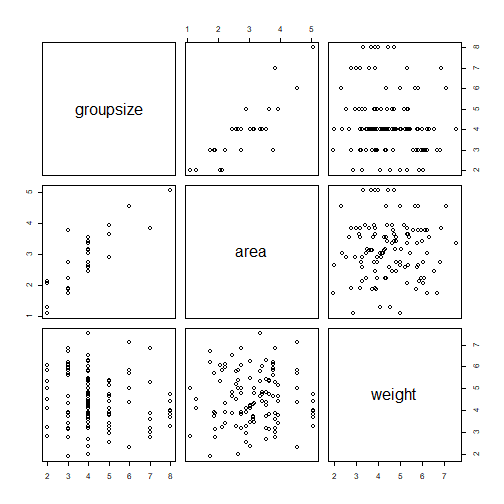### Practice Question 5H3

Finally, consider the avgfood variable. Fit two more multiple regressions: (1) body weight as an additive function of avgfood and groupsize, and (2) body weight as an additive function of all three variables, avgfood and groupsize and area. Compare the results of these models to the previous models you’ve fit, in the first two exercises. (a) Is avgfood or area a better predictor of body weight? If you had to choose one or the other to include in a model, which would it be? Support your assessment with any tables or plots you choose. (b) When both avgfood or area are in the same model, their effects are reduced (closer to zero) and their standard errors are larger than when they are included in separate models. Can you explain this result?

First, let’s estimate the model with average food and group size.

m <- map(
alist(
weight ~ dnorm(mu, sigma),
mu <- a + bf * avgfood + bg * groupsize,
a ~ dnorm(5, 5),
bf ~ dnorm(0, 5),
bg ~ dnorm(0, 5),
sigma ~ dunif(0, 5)
),
data = d
)
precis(m)

##        Mean StdDev  5.5% 94.5%
## a      4.18   0.43  3.50  4.86
## bf     3.60   1.18  1.72  5.48
## bg    -0.54   0.15 -0.79 -0.30
## sigma  1.12   0.07  1.00  1.23


This model shows a large positive relationship between average food and body weight and a negative relationship between group size and body weight. The estimate for the group size slope is similar to that in the model with both territory area and group size. Thus, the masking effect on group size was overcome by including the average food variable. Perhaps average food and territory size are positively correlated (to have similar effects).

Now we can estimate the model with three predictors.

m <- map(
alist(
weight ~ dnorm(mu, sigma),
mu <- a + bf * avgfood + bg * groupsize + ba * area,
a ~ dnorm(5, 5),
bf ~ dnorm(0, 5),
bg ~ dnorm(0, 5),
ba ~ dnorm(0, 5),
sigma ~ dunif(0, 5)
),
data = d
)
precis(m)

##        Mean StdDev  5.5% 94.5%
## a      4.10   0.42  3.42  4.78
## bf     2.30   1.39  0.08  4.53
## bg    -0.59   0.15 -0.84 -0.35
## ba     0.40   0.24  0.02  0.78
## sigma  1.10   0.07  0.99  1.22


This model is similar to the previous one in terms of the group size slope, so we have again addressed the masking relationship problem. However, the estimates of the average food and territory area slopes have decreased in magnitude compared to previous models. This is likely due to multicollinearity between the two variables.

In response to question (a), I think average food and territory area are both fine predictors of body weight. However, given their high positive correlation with one another and similar relationships with body weight, I would not include both in the same model. To select between them, I might favor the one with the larger standardized slope estimate.

d$avgfood.s <- (d$avgfood - mean(d$avgfood)) / sd(d$avgfood)
m <- map(
alist(
weight ~ dnorm(mu, sigma),
mu <- a + bf * avgfood.s + bg * groupsize,
a ~ dnorm(5, 5),
bf ~ dnorm(0, 5),
bg ~ dnorm(0, 5),
sigma ~ dunif(0, 5)
),
data = d
)
precis(m)

##        Mean StdDev  5.5% 94.5%
## a      6.96   0.68  5.87  8.04
## bf     0.75   0.24  0.36  1.13
## bg    -0.56   0.15 -0.81 -0.31
## sigma  1.12   0.07  1.00  1.23

d$area.s <- (d$area - mean(d$area)) / sd(d$area)
m <- map(
alist(
weight ~ dnorm(mu, sigma),
mu <- a + ba * area.s + bg * groupsize,
a ~ dnorm(5, 5),
ba ~ dnorm(0, 5),
bg ~ dnorm(0, 5),
sigma ~ dunif(0, 5)
),
data = d
)
precis(m)

##        Mean StdDev  5.5% 94.5%
## a      6.39   0.53  5.54  7.24
## ba     0.57   0.18  0.27  0.86
## bg    -0.43   0.12 -0.62 -0.24
## sigma  1.12   0.07  1.00  1.24


Given these results, it looks like average food would be preferable to territory area.

In response to question (b), this result is likely due to multicollinearity between average food and territory area. The two variables are highly correlated and have very similar relationships with body weight. Thus, the partial effect of each becomes smaller (when controlling for the other).

### Session Info

sessionInfo()

## R version 3.5.1 (2018-07-02)
## Platform: x86_64-w64-mingw32/x64 (64-bit)
## Running under: Windows >= 8 x64 (build 9200)
##
## Matrix products: default
##
## locale:
##  LC_COLLATE=English_United States.1252
##  LC_CTYPE=English_United States.1252
##  LC_MONETARY=English_United States.1252
##  LC_NUMERIC=C
##  LC_TIME=English_United States.1252
##
## attached base packages:
##  parallel  stats     graphics  grDevices utils     datasets  methods
##  base
##
## other attached packages:
##  knitr_1.21           RWordPress_0.2-3     rethinking_1.59
##  usethis_1.4.0
##
## loaded via a namespace (and not attached):
##   tidyselect_0.2.5   xfun_0.4           purrr_0.2.5
##   lattice_0.20-35    colorspace_1.3-2   htmltools_0.3.6
##   stats4_3.5.1       loo_2.0.0          yaml_2.2.0
##  base64enc_0.1-3    XML_3.98-1.16      rlang_0.3.0.1
##  pkgbuild_1.0.2     pillar_1.3.1       glue_1.3.0
##  withr_2.1.2        bindrcpp_0.2.2     matrixStats_0.54.0
##  bindr_0.1.1        plyr_1.8.4         stringr_1.3.1
##  munsell_0.5.0      gtable_0.2.0       mvtnorm_1.0-8
##  coda_0.19-2        evaluate_0.12      inline_0.3.15
##  callr_3.1.1        ps_1.3.0           highr_0.7
##  XMLRPC_0.3-1       Rcpp_1.0.0         scales_1.0.0
##  jsonlite_1.6       fs_1.2.6           gridExtra_2.3
##  digest_0.6.18      stringi_1.2.4      processx_3.2.1
##  dplyr_0.7.8        grid_3.5.1         bitops_1.0-6
##  cli_1.0.1          tools_3.5.1        magrittr_1.5
##  RCurl_1.95-4.11    lazyeval_0.2.1     tibble_1.4.2
##  crayon_1.3.4       pkgconfig_2.0.2    MASS_7.3-50
##  rsconnect_0.8.12   prettyunits_1.0.2  assertthat_0.2.0
##  rmarkdown_1.11     rstudioapi_0.8     R6_2.3.0
##  compiler_3.5.1


### References

McElreath, R. (2016). Statistical rethinking: A Bayesian course with examples in R and Stan. New York, NY: CRC Press.

### 2 Responses to “Statistical Rethinking: Chapter 5 Practice”

1.2.In case of 5E1, $μ_i = α + β(x_i − z_i)$ is not multiple regression model as we are regressing on a single variable which happens to be the difference of two variables.